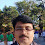# Ionic Conduction-10th Science Learning Cards

Chapter-22

Ionic Conduction

Learning Card-1

1. Write any two examples for metallic conductors.

Iron, Aluminium

2. Name the cation and Anion in Common salt.

Cation = Na+ Anion = Cl-

3. What is an electrolyte?

The Chemical substances that conduct electricity in aqueaus state are called Electrolytes

4. Give two example for strong electrolyte.

Sodium chloride, Copper sulphate

5. Define electrolysis.

The chemical reaction caused by electricity is called electrolysis.

6. What is electroplating? Write any two uses.

The process of depositing a layer of any desired metal on another metallic object by means of electricity is called electroplating.

- It improves the appearance

- It prevents the corrosion

7. Write any two electrolytic Conductors.

Sodium hydroxide, hydrochloric acid.

8. Chemical equivalent of substance is given by

a) Atomic mass X valency

b) Atomic mass + valency

c) Atomic mass / valency

d) Atomic mass – valency

c) Atomic mass / valency

9. Write a neat diagram of voltmeter and label the parts.

10. Write a neat diagram of electroplating and lable the parts.

11. What is the value of Faraday’s Constant

F= 96,500 Coulomb/mole

Chapter-22

Ionic Conduction

Learning Card-2

1. What is an Ion? Name the two types

Charged atoms are called ions.

Cation and anion

2. What is electrochemical equivalent?

Electrochemical Equivalent is defined as the mass in grams liberated by unit current in unit time

3. Write the relation between ECE and mass of the substance

4. Differentiate metallic conduction and electrolytic conduction. Give examples.

 Metallic conduction Electrolytic Conduction Physical change Chemical change Only electrons conduct electricity Positive as well as negative ions conduct electricity All metals and metallic alloys show metallic consuction Ionic compounds show electrolytic conduction in equeous solution

5. Differentiate strong and weak electrolytes. Give examples.

 Strong electrolyte Weak electrolyte Complete dissociation in aqueous solution Ex : Nacl Partial dissociation in aqueous solution Ex : CH3COOH

6. Write Faraday’s laws of electrolys’s

First law: The mass of substance deposited or collected at either electrodes during electrolysis is proportional to the current and to the time.

Second law: The mass of different substances liberated by the same quantity of elecricity are propotional to their chemical equivalent

7. Sea water is a good conductor of electricity than drinking water. Why?

Sea water contains sodium, chloride it converts seawater into electrodes, that’s why it conduct electricity

8. Write four applications of electrolysis

1) Electroplating

2) Electrotyping

3) Purification of metal

4) Electro polishing.

9. During electrolysis of water, gas liberated at Anode is

a) Carbondioxide b) Oxygen c) Nitrogen d) Hydrogen

b) Oxygen

10. Does pure water conduct electricity? If not why?

No. Pure water is non electrolyte.

Chapter-22

Ionic Conduction

Learning Card-3

1. Define electrolysis with an example.

The chemical reaction caused by electricity is called electrolysis.

Ex. Dissociation of copper-sulphate.

2. Explain Arrehenius theory of Dessociation.

Arrhenius proposed the theory of slutrytfe dissociation. According to this theory the molecules of an electrolyte in aqueans solution breaks into ions and trelly more about in the bulk of the solution.

3. ECE of gold is 0.0006812. What do you mean by this?

It means 0.0006812 of gold is deposited when 1 amphre current is made flow in a solution for 1 second.

4. How long should a current of 3 Ampere be applred through a soulution of silvernitrate to coat a metal surface of 80cm2 with 0.005cm thick layers? Density of silver is 10.59/cm2

5. What mass copper could be deposited from a copper II. Sulphate solution using a current of 0.50 A over 10 seconds?

Calculate the quantity of electricity

Q = I x t

I = 0.50A

T = 10 Seconds

Q = 0.50 x 10 = 5.0

6. How much time is required to deposit 1.10g of copper by passing 0.5 Ampere of current? (If ECE of Cu is 0.00033g/colum)

z = 0.00033

T =?

I = 0.5 ampere

M = 1.10g

M=Zlt

t=M/Zl

t=1.10/(0.00033 X 0.5)

t=6666.666 seconds

7. Sodium chloride conducts electricity in fused state why?

In fused state, the electrostatic forces between the sodium and chloride icons are weakened; such that ions can migrate towards oppositely- charged electrodes, and therefore fused sodium chloride conducts electric current

8. Compare the flow of electricity through copper metal and copper sulphate solution.

A metal conducts electric current due to the migration of electrons where as electrolyte conducts electric current due to the migration and discharge of cations and atoms

9. How do you account for loss of copper from solution during electroplatig of copper?

From the other plate an equal amount of copper gets dis charged in the solution. Thus the loss of copper from the solution gets compensated and the process keeps going, this means that copper gets transferred from one plate to another.

10. Write chemical change occurred at Anode and Cathode during electrolysis of CuCl2

On platinum cathode, a reddish layer of copper is deposited

Cu2 + 2c- --> Cu

On carbon anode, carbon dioxide gas is formed due to the discharge of OH- ionsYou Might Like

1.